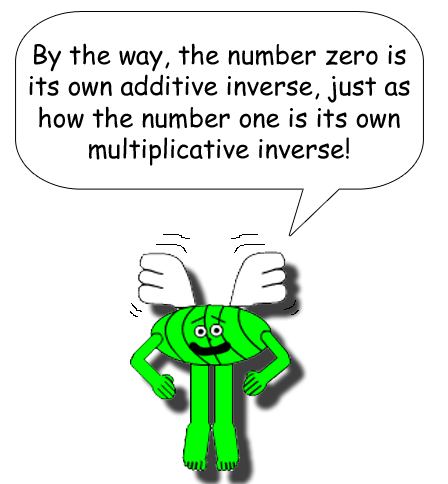# Super Summation 2

## A summation will always give you zero if the number above the summation sign (sigma) & the one below are additive inverses!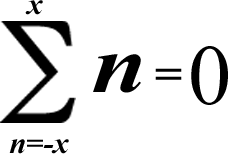(Of course the negative number always has to be below the sigma!)

## Examples:(-3) + (-2) + (-1) + 0 + 1 + 2 + 3 = 0

[All of the additive inverses cancel each other out & leave you with zero!]

### The negative numbers are put in parentheses to prevent confusion with addition or subtraction.(-7) + (-6) + (-5) + (-4) + (-3) + (-2) + (-1) + 0 + 1 + 2 + 3 + 4 + 5 + 6 + 7 = 0

[All of the additive inverses cancel each other out & leave you with zero!]

## If you don't pick the right additive inverse, you won't get zero; however, something else happens...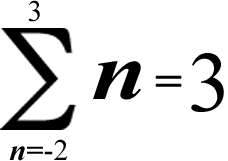(-2) + (-1) + 0 + 1 + 2 + 3 = 0 + 3 = 3

[The additive inverse of 3 was excluded this time, so you had to add 3 to zero, which of course gives you 3]If you can see her, this character's name is Miss Zero Digit.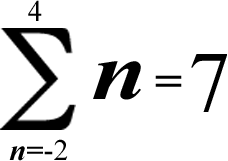(-2) + (-1) + 0 + 1 + 2 + 3 + 4 = 3 + 4 = 7

[The additive inverses of 3 & 4 were excluded; the positive numbers added up to 7]

If you exclude more than 1 additive inverse, the remaining positive or negative numbers will add up to their respective sums!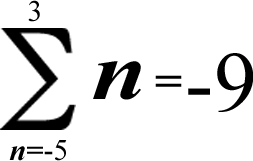(-5) + (-4) + (-3) + (-2) + (-1) + 0 + 1 + 2 + 3 = (-5) + (-4) = -9

[The additive inverses of -5 & -4 were excluded; the negative numbers added up to -9]

If you have more positive integers left, the sum will be positive; if you have more negative integers left, the sum will be negative. In other words, the greater absolute value will reign supreme!